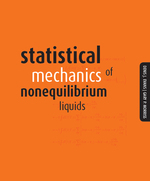# Statistical Mechanics of Nonequilibrium Liquids

DENIS J. EVANS
GARY P. MORRISS
https://www.jstor.org/stable/j.ctt24h99q

1. Front Matter
(pp. i-iv)
(pp. v-viii)
3. Preface
(pp. ix-x)
D. J. Evans and G. P. Morriss
4. Biographies
(pp. xi-xii)
5. List of Symbols
(pp. xiii-xvi)
6. 1. Introduction
(pp. 1-10)

Mechanics provides a complete microscopic description of the state of a system. When the equations of motion are combined with initial conditions and boundary conditions, the subsequent time evolution of a classical system can be predicted. In systems with more than just a few degrees of freedom such an exercise is impossible. There is simply no practical way of measuring the initial microscopic state of for example, a glass of water, at some instant in time. In any case, even if this was possible we could not then solve the equations of motion for a coupled system of 1023 molecules....

7. 2. Linear Irreversible Thermodynamics
(pp. 11-32)

At the hydrodynamic level we are interested in the macroscopic evolution of densities of conserved extensive variables such as mass, energy and momentum. Because these quantities are conserved, their respective densities can only change by a process of redistribution. As we shall see, this means that the relaxation of these densities is slow, and therefore the relaxation plays a macroscopic role. If this relaxation were fast (i.e. if it occurred on a molecular time scale for instance) it would be unobservable at a macroscopic level. The macroscopic equations of motion for the densities of conserved quantities are called the Navier-Stokes...

8. 3. The Microscopic Connection
(pp. 33-76)

In nonequilibrium statistical mechanics we seek to model transport processes beginning with an understanding of the motion and interactions of individual atoms or molecules. The laws of classical mechanics govern the motion of atoms and molecules so in this chapter we begin with a brief description of the mechanics of Newton, Lagrange and Hamilton. It is often useful to be able to treat constrained mechanical systems. We will use a Principle due to Gauss to treat many different types of constraint - from simple bond length constraints, to constraints on kinetic energy. As we shall see, kinetic energy constraints are...

9. 4. The Green Kubo Relations
(pp. 77-92)

In 1828 the botanist Robert Brown observed the motion of pollen grains suspended in a fluid. Although the system was allowed to come to equilibrium, he observed that the grains seemed to undergo a kind of unending irregular motion. This motion is now known as Brownian motion. The motion of large pollen grains suspended in a fluid composed of much lighter particles can be modelled by dividing the accelerating force into two components: a slowly varying drag force, and a rapidly varying random force due to the thermal fluctuations in the velocities of the solvent molecules. The Langevin equation as...

10. 5. Linear Response Theory
(pp. 93-116)

In this chapter we will discuss how an external field Fe, perturbs an N-particle system. We assume that the field is sufficiently weak that only the linear response of the system need be considered. These considerations will lead us to equilibrium fluctuation expressions for mechanical transport coefficients such as the electrical conductivity. These expressions are formally identical to the Green-Kubo formulae that were derived in the last chapter. The difference is that the Green-Kubo formulae pertain to thermal transport processes where boundary conditions perturb the system away from equilibrium - all Navier-Stokes processes fall into this category. Mechanical transport coefficients...

11. 6. Computer Simulation Algorithms
(pp. 117-166)

We will now show how linear response theory can be used to design computer simulation algorithms for the calculation of transport coefficients. There are two types of transport coefficients: mechanical and thermal. In this chapter we will show how thermal transport coefficients can be calculated using mechanical methods.

In nature nonequilibrium systems may respond essentially adiabatically, or depending upon circumstances, they may respond in an approximately isothermal manner - the quasi-isothermal response. No natural systems can be precisely adiabatic or isothermal. There will always be some transfer of the dissipative heat produced in nonequilibrium systems towards thermal boundaries. This heat...

12. 7. Nonlinear Response Theory
(pp. 167-208)

In Chapter 6 we saw that nonequilibrium molecular dynamics leads inevitably to questions regarding the nonlinear response of systems. In this chapter we will begin a discussion of this subject.

It is not widely known that in Kubo's original 1957 paper (Kubo, 1957), he not only presented results for adiabatic linear response theory, but that he also included a formal treatment of the adiabatic nonlinear response. The reason why this fact is not widely known is that, like many treatments of nonlinear response theory that followed, his formal results were exceedingly difficult to translate into a useful, experimentally verifiable forms....

13. 8. Time Dependent Response Theory
(pp. 209-230)

In this chapter we extend the nonlinear response theory discussed in Chapter 7 to describe the response of classical, many-body systems to time dependent external fields. The resulting formalism is applicable to both adiabatic and thermostatted systems. The results are then related to a number of known special cases: time dependent linear response theory, and time independent nonlinear response theory as described by the transient time correlation approach and the Kawasaki response formula.

We begin by developing a formal operator algebra for manipulating distribution functions and mechanical phase variables in a thermostatted system subject to a time dependent applied field....